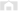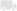# How to calculate square metres# How to calculate square metres

×

## Project Overview

Calculating the size of a space, as accurately as possible, is an important step in any construction or D.I.Y. project. You need these measurements to be right when ordering materials. Follow the simple steps in this video to make sure you’re measuring square metres correctly.
Continue to step-by-step instructions

## Step by Step Instructions

1 Break down your area for measuring
3 Use your measurements to calculate the area
• ## Step 1. Break down your area for measuring

When measuring large areas, breaking it down into smaller sections will make it easier. For this project, we have broken our space into two rectangles that will be measured separately then added together. Measure the first of these rectangles, starting with the width, followed by the length, and repeat the process on the second rectangle. Mark these measurements in your notebook.
• ## Step 2. Round up your measurements

It’s always a good idea to round up your measurements. Once you have finished calculating all the measurements, add a 10 per cent contingency on top to make sure you have more than enough materials for the job you’re about to undertake.

• ## Step 3. Use your measurements to calculate the area

To calculate the area of each of your rectangles, multiply the length by the width. For this project, that sum is 3.3m x 1.3m = 3.63m2 for the first rectangle, and 2.6 x 5.2 = 13.52m2 for the second. Because we are measuring the whole deck, we add these numbers together to get the final area measurement. So that sum is 3.63 + 13.52 = 17.15m2. Make sure to add the 10 per cent contingency to this final sum. You can do this by multiplying the total by 1.1. So 17.15 x 1.1 = 18.865m2.

## Tools and Materials

• Calculator
• Pencil
• Tape measure

### Materials